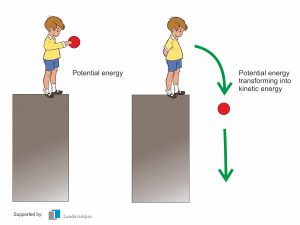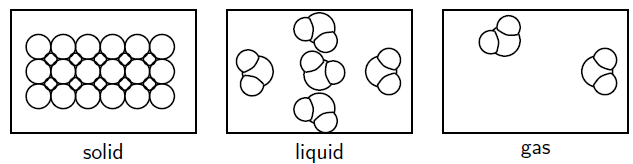# Kinetic Molecular Theory Study Guide

INTRODUCTION

Recognize these crazy balls that made our childhood so much fun? The harder you threw it, the more it would bounce. The science behind this is pretty simple. The harder you throw the ball, the greater the kinetic energy you provide to the ball, resulting in greater velocity and eventually more bounce. Similar is the concept of the kinetic molecular theory in chemistry, that we are about to discuss.### KINETIC MOLECULAR THEORY

The kinetic molecular theory explains how microscopic properties of atoms and their interactions can be observed at the macroscopic level (like pressure, volume, temperature), as well as their interaction with one another. Using the concept, we may be able to explain why matter consists of various stages and how matter can change between them.

According to the kinetic molecular theory of matter:

• The matter is made up of particles that are constantly moving.
• Every particle has energy, however the amount of energy changes based on the temperature of the matter specimen. The condition of the material is then determined by whether it is solid, liquid, or gaseous. Particles in the solid-state have the minimum amount of energy, whereas gaseous particles have the most.
• The average kinetic energy of the molecules is measured by the temperature of a material.
• Whenever the energy of the molecules is modified, the phase of the particles may change.
• There is space between matter particles. As a particular substance travels from the solid to the liquid and gaseous phases, the average amount of vacant space between molecules rises.
• Molecular interactions become stronger when molecules draw closer together due to attractive forces between them. These forces of attraction are called forces between molecules.

The kinetic molecular theory is easiest to apply to gasses. Every gas molecule is thought to be self-contained and unaffected by the presence of other gas particles. When a gas particle collides with another gas molecule, it simply bounces off in the opposite direction without any speed change. The same logic applies to hot air balloons. As air particles heat up and rise, they collide with the interior of the balloon, propelling it higher into the air.### Applying Kinetic Theory to Gas Laws

Particles of a gas are dimensionless points in random motion. Particles don’t interact apart from plastic collisions bouncing off one another. These are called ideal gases. To examine an ideal gas we have to discuss four variables.

• Pressure (P): This is the force the gas is exerting on its container.
• Temperature (T): This is the amount of heat energy available to be transferred into kinetic energy of motion.
• Volume (V): How big is the container?
• Moles (n): How many particles are there in the container?

These variables are dependent on each other in interesting ways that have been formulated into laws.

1. Boyles Law

Pressure and volume of gas at constant temperature relates that its pressure is inversely proportional to its volume or the product of pressure and volume is constant. PV = Constant. For two states of gas we can use P1V1 = P2V2 if T is constant.

__2. Charles Law __

Volume and temperature are directly proportional V α T if one doubles the other must double. When we do calculations with temperature we must always use an absolute temperature scale called the Kelvin scale.

__3. Combined Gas Law __

the combined gas law is like a combination of Boyles and Charles law. P1V1/T1 = P2V2/T2

Equal volumes of gases at the same temperature and pressure contain same number of molecules. Specifically one mole of ideal gas occupies 22.4 liters at standard temperature and pressure regardless of the identity of the gas.

5. Ideal Gas Law

All the variables co relate in one equation called the ideal gas law PV = nRT. This also contains an ideal gas constant R.

### CONCLUSION

• The kinetic molecular theory of matter describes the microscopic properties of atoms and their interrelations.
• The average kinetic energy of the molecules is measured by the temperature of a material.
• Whenever the energy of the particulate is modified, the phase of the particles may shift.

Learn about atoms in depth: Atoms Study Guide

### FAQs:

1. What are some examples of kinetic molecular theory?

Brownian Motion—the random movement of particulate matter caused by collisions with “air” molecules, and Boyle’s, Charles’, and Gay- Lussac’s Laws—are examples of kinetic theory. This theory also emphasizes how temperature influences the states of matter.

2. What is the application of kinetic molecular theory?

The kinetic molecular theory may explain each of the empirically established gas laws. The pressure of a gas is caused by clashes between the gas molecules and the container’s surfaces. When a gas particle collides with a wall, it generates a force on it.

3. What is the kinetic theory of matter? Explain it with an example.

According to the kinetic molecular theory of matter, the matter is composed of continually moving particles. Every particle has energy, however the amount of energy changes based on the temperature of the matter specimen. The condition of the material is then determined by whether it is solid, liquid, or gaseous.

We hope you enjoyed studying this lesson and learned something cool about Kinetic Molecular Theory! Join our Discord community to get any questions you may have answered and to engage with other students just like you! Don’t forget to download our app to experience our fun VR classrooms – we promise it makes studying much more fun! 😎

]]>